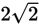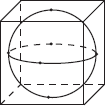# SAT Math Multiple Choice Question 984: Answer and Explanation

### Test Information

Question: 984

4. What is the radius of the largest sphere that can be placed inside a cube that hasa volume of 64 cubic units?

• A. 2
• B.• C. 4
• D. 8

Explanation:

A

Difficulty: Medium

Category: Additional Topics in Math / Geometry

Strategic Advice: Often, a verbal description of a geometric scenario is simply not enough to answer a question. Instead, you need to draw a quick sketch to visualize the situation.

Getting to the Answer: The figure below shows the sphere inside the cube.The sphere will touch the cube at six points. Each point will be an endpoint of a diameter and will be at the center of one of the cube's faces. Therefore, the diameter of the sphere extends directly from one face of the cube to the opposite face and is perpendicular to both faces that it touches. This means that the diameter must have the same length as an edge of the cube. The volume of the cube is given (64), so express this as s3 = 64, and take the cube root of 64 to find that the length of each edge is 4. The diameter of the sphere is therefore also 4, so its radius must be 2, making (A) the correct answer.